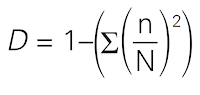>

## 01 July 2016

### #146 Assessing species diversityThere are 2 types of sampling: random sampling and systematic sampling.

Random sampling

• should be used when an area looks reasonably uniform
• there is no clear pattern to the way the species are distributed

--> avoids bias- a square frame that marks off area of ground or water
- used to identify the different species present and/or to measure abundance
- use results to calculate:

• species frequency: measure of chance of a particular species being found within any one quadrat
• species density: measure of how many individuals there are per unit area
- use results to estimate:
• percentage cover: the percentage of area inside the quadrat that is occupied by each species
• abundance scale (e.g.: Braun-Blanquet scale) for number and plant cover

b) Mark-release-recapture: estimating the population size of mobile organisms
1. As many individuals caught as possible
2. Individuals get marked (in a way that will not affect its future chances of survival)
3. Marked individuals are counted (a)
4. Marked individuals are returned to their habitats to mix randomly with their population
5. After enough time has elapsed (at least 24 hours, maximum __ hours), a large sample is recaptured
6. Number of marked (b) and unmarked individuals are counted
|_____________________|
|
(c)

Systematic sampling

• investigate species distribution where physical conditions change
• e.g.: altitude, soil moisture content, soil pH, exposure/ light intensity
• record identity of organisms that touch the line at set distances
• data shown as a drawing

b) Belt transect
• place quadrats at regular intervals along line  --> record abundance of species within quadrat
• data plotted as bar chart or kite diagram

Correlation - plot a scatter graph or calculate correlation coefficient (r)

*strength of correlation = how close the points are to the straight line

1. Pearson's correlation coefficientuse with 2 sets of data when data:

• continuous data has been collected
• must be normally distributed
• may be a linear correlation (draw scatter diagram first)
• quantitative data collected as measurements/counts
• number of paired observations is at least 5, ideally 10 or more

2. Spearman's rank correlation
use when data:

• data points are independent of each other
• data is correlated, but not linear (draw scatter diagram first)
• number of paired observations: at least 5, ideally between 10 and 30

- rank data for each variable and assess the difference between the rankings
- make a null hypothesis

Simpson's Index of Diversity

When you have  collected information about the abundance of he species in the are you are studying, use the results to calculate a value for species diversity in that area

 Syllabus 2016-2018 18.1 Biodiversity Biodiversity is much more than a list of all the species in a particular area. a) define the terms species, ecosystem and niche  b) explain that biodiversity is considered at three different levels:  • variation in ecosystems or habitats  • the number of species and their relative abundance  • genetic variation within each species  c) explain the importance of random sampling in determining the biodiversity of an area  d) use suitable methods, such as frame quadrats, line transects, belt transects and mark-release-recapture, to assess the distribution and abundance of organisms in a local area  e) use Spearman’s rank correlation and Pearson’s linear correlation to analyse the relationships between the distribution and abundance of species and abiotic or biotic factorsf) use Simpson’s Index of Diversity (D) to calculate the biodiversity of a habitat and state the significance of different values of D